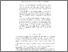# A scattering of orders

Abraham, Uri, Bonnet, Robert, Cummings, James, Džamonja, Mirna and Thompson, Katherine (2012) A scattering of orders. Transactions of the American Mathematical Society, 364. pp. 6259-6278. ISSN 0002-9947Preview PDF (Accepted manuscript) - Accepted Version Download (252kB) | Preview

## Abstract

A linear ordering is scattered if it does not contain a copy of the rationals. Hausdorff characterised the class of scattered linear orderings as the least family of linear orderings that includes the class $\mathcal B$ of well-orderings and reversed well-orderings, and is closed under lexicographic sums with index set in $\mathcal B$. More generally, we say that a partial ordering is $\kappa$-scattered if it does not contain a copy of any $\kappa$-dense linear ordering. We prove analogues of Hausdorff's result for $\kappa$-scattered linear orderings, and for $\kappa$-scattered partial orderings satisfying the finite antichain condition. We also study the $\mathbb{Q}_\kappa$-scattered partial orderings, where $\mathbb{Q}_\kappa$ is the saturated linear ordering of cardinality $\kappa$, and a partial ordering is $\mathbb{Q}_\kappa$-scattered when it embeds no copy of $\mathbb{Q}_\kappa$. We classify the $\mathbb{Q}_\kappa$-scattered partial orderings with the finite antichain condition relative to the $\mathbb{Q}_\kappa$-scattered linear orderings. We show that in general the property of being a $\mathbb{Q}_\kappa$-scattered linear ordering is not absolute, and argue that this makes a classification theorem for such orderings hard to achieve without extra set-theoretic assumptions.

Item Type: Article Faculty of Science > School of Mathematics Faculty of Science > Research Groups > Logic Vishal Gautam 18 Mar 2011 10:19 23 Oct 2022 00:08 https://ueaeprints.uea.ac.uk/id/eprint/19975 10.1090/S0002-9947-2012-05466-3View Item